International Journal of Applied Mathematics and Theoretical Physics
Volume 1, Issue 2, July 2015, Pages: 14-18

Methodology Article

Using Polynomial Regression to Objectively Test the Fit of Calibration Curves in Analytical Chemistry

Seth H. Frisbie1, 2, *, Erika J. Mitchell2, Kenneth R. Sikora1, Marwan S. Abualrub3,
Yousef Abosalem3

1Department of Chemistry and Biochemistry, Norwich University, Northfield, Vermont, USA

2Better Life Laboratories, Inc., East Calais, Vermont, USA

3Department of Mathematics/Preparatory Program, Khalifa University, Abu Dhabi, United Arab Emirates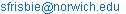(S. H. Frisbie)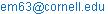(E. J. Mitchell)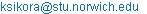(K. R. Sikora)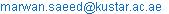(M. S. Abualrub)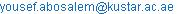(Y. Abosalem)

Seth H. Frisbie, Erika J. Mitchell, Kenneth R. Sikora, Marwan S. Abualrub, Yousef Abosalem. Using Polynomial Regression to Objectively Test the Fit of Calibration Curves in Analytical Chemistry. International Journal of Applied Mathematics and Theoretical Physics. Vol. 1, No. 2, 2015, pp. 14-18. doi: 10.11648/j.ijamtp.20150102.11

Abstract: Calibration curves are commonly used for quantitative analysis in analytical chemistry to calculate the concentrations of chemicals in samples. Typically, the concentration of the analyte, the chemical being quantified, is the independent variable and is plotted on the x-axis. The detector response, the reading from the instrument, is the dependent variable and is plotted on the y-axis. A calibration curve is made by plotting the known concentration of analyte versus the detector response. After a calibration curve is made, the unknown concentration of analyte in any sample is calculated from its detector response. Unfortunately, there is no standard procedure for objectively testing the fit of calibration curves in analytical chemistry. For example, the World Health Organization (WHO) and the United States Environmental Protection Agency (U.S. EPA) do not provide guidance for testing the linearity or curvature of calibration curves. Moreover, this important topic is not broached in at least 5 of the leading analytical chemistry textbooks. However, there is a simple and effective way to fix this deficiency. In this paper, the use of polynomial regression to objectively test the fit of calibration curves in drinking water analysis is demonstrated. Polynomial regression was used to test the linearity of a representative calibration curve for the spectrophotometric determination of arsenic in drinking water by the arsenomolybdate method. And polynomial regression was used to test the curvature of a representative calibration curve for the determination of arsenic in drinking water by graphite furnace atomic absorption spectroscopy. Microsoft® Excel® 2010 and 2016, MiniTab® 17.2.1, and RStudio® 0.99.441 were used to calculate these calibration curves; in all cases, the calibration curves from these 3 programs agreed with each other to at least 3 significant figures.

Keywords: Polynomial Regression, Calibration Curve, Analytical Chemistry

Contents

1. Introduction

The World Health Organization (WHO) and the United States Environmental Protection Agency (U.S. EPA) do not provide guidance for testing the linearity of calibration curves [1, 2]. As a result, drinking water laboratories do not have a standard test for this linearity. Fortunately, polynomial regression provides a simple, reliable, and objective test for the linearity of calibration curves [3, 4].

2. Materials and Methods

Six standard solutions were analyzed for total arsenic (As) by the arsenomolybdate method with a Jenway 6305 spectrophotometer . These 6 standard solutions contained 0, 14, 28.6, 57.1, 114, and 229 micrograms of arsenic per liter of deionized water, respectively (Table 1).

Similarly, 6 standard solutions were analyzed for total arsenic by graphite furnace atomic absorption spectroscopy with a Buck Scientific 220AS autosampler, 220GF graphite furnace, and 210VGP atomic absorption spectrometer . These 6 standard solutions contained 0, 1.0, 5.0, 15.0, 30.0 and 50.0 micrograms of arsenic per liter of deionized water, respectively (Table 2). A 1.00 milliliter aliquot of each standard solution was loaded onto the autosampler. The matrix of each 1.00 milliliter aliquot was modified with 50.0 microliters of 10.0% (weight/volume) ammonium nitrate (NH4NO3) in deionized water, 50.0 microliters of 0.2% (weight/volume) palladium nitrate (Pd(NO3)2) in 2% (weight/volume) nitric acid (HNO3), and 50.0 microliters of 1.79% (weight/volume) magnesium nitrate hexahydrate (Mg(NO3)2·6H2O) in deionized water. The autosampler delivered a 20.0 microliter aliquot of this mixture to the graphite furnace. The furnace tube was made from nonpyrolytic graphite. The furnace initialized at 100°C (Celsius) for 10 seconds, heated to 250°C for 20 seconds, dried the mixture at 250°C for 15 seconds, heated to 750°C for 25 seconds, ashed the mixture at 750°C for 10 seconds, heated to 2,200°C for 1.5 seconds, and atomized the mixture at 2,200°C for 3 seconds. The sheath and internal flows of argon (Ar) gas were 1,200 and 200 milliliters per minute, respectively. The absorbance from a hollow-cathode lamp was read at 193.7 nanometers through a 0.7 nanometer slit and after deuterium (D2) background correction. This absorbance was measured for 2.4 seconds during atomization. Finally, this absorbance over time was used to calculate arsenic concentration [5, 6, 3].

All of the statistics in this research paper were calculated using Microsoft® Excel® 2010 and 2016, MiniTab® 17.2.1, and RStudio® 0.99.441.

3. Results and Discussion

A.  Using Polynomial Regression to Test the Fit of Linear Calibration Curves.

If a linear calibration curve is expected, then a regression of detector response on the concentration of analyte and the concentration of analyte squared is used to test the significance of a second-order or quadratic effect. This test assumes that there are no systematic errors; that is, this test assumes that all errors are random . If this quadratic effect is statistically significant at α = 0.05, the calibration curve is not linear and 1 or more systematic errors may need to be corrected. If this quadratic effect is not statistically significant, the calibration curve is linear and the null hypothesis that the y-intercept goes through the origin is tested. At least 4 different concentrations of standard solution are required for this test of linearity.

Calibration results from the spectrophotometric determination of arsenic in drinking water by the arsenomolybdate method are used to evaluate this test of linearity  (Table 1). Beer’s law says a linear calibration curve with the y-intercept going through the origin (0, 0) is expected ; therefore, a regression of absorbance on the concentration of arsenic and the concentration of arsenic squared is used to test the significance of a quadratic effect. The results of this first regression are shown in Equation 1.

(Equation 1) Absorbance = −4.02x10−8(µg/L)2 + 0.00169(µg/L) + 0.00138

The quadratic effect coefficient (−4.02x10−8) is equivalent to 0 at the 95% confidence level. It has a 95% confidence interval that ranges from −1.09x10−6 to 1.01x10−6. This conclusion agrees with Beer’s law and does not suggest the presence of systematic error. Therefore, the quadratic term is removed from the model and a regression of absorbance on the concentration of arsenic is used to test the significance of a linear effect. The results of this second regression are shown in Equation 2.

(Equation 2) Absorbance = 0.00168(µg/L) + 0.00159

The linear effect coefficient (0.00168) is different from 0 at the 95% confidence level. It has a 95% confidence interval that ranges from 0.00163 to 0.00173. Therefore, the linear term is used in the model and the null hypothesis that the y-intercept goes through the origin is tested. The y-intercept (0.00159) is equivalent to 0 at the 95% confidence level. It has a 95% confidence interval that ranges from −0.00371 to 0.00690. These 2 conclusions agree with Beer’s law and do not suggest the presence of systematic error. Therefore, the y-intercept is removed from the model and linear regression through the origin is used for the calibration equation. The results of this third and final regression are shown in Equation 3.

(Equation 3) Absorbance = 0.00169(µg/L)

In summary, this calibration obeys Beer’s law (Equation 3; Figure 1). That is, the plot of absorbance versus arsenic concentration is linear and the y-intercept goes through the origin.Figure 1. Calibration curve for the determination of total arsenic in drinking water by the arsenomolybdate method. Concentration is the independent or x variable. The units of concentration are micrograms of arsenic per liter of deionized water (µg/L). Absorbance is the dependent or y variable.

It is very important to test if the y-intercept goes through the origin for the following reasons. First, theory predicts an analyte concentration of 0 gives a detector response of 0. Second, regression through the origin increases accuracy and precision when samples have analyte concentrations at or near the limit of detection. Third, this test can help identify contaminated blanks and standards. Fourth, this test can help identify inadequate or failed background correction in atomic absorption, atomic emission, atomic fluorescence, and inductively coupled plasma spectroscopy.

In addition, polynomial regression can be used to measure the linear range of a calibration curve. If a linear calibration curve is expected, then increasingly concentrated standard solutions are analyzed until a significant quadratic effect is observed. At this point the detector is saturated. The linear range starts at the limit of detection and ends at the most concentrated standard that gives a linear calibration curve.

B.  Using Polynomial Regression to Test the Fit of Quadratic Calibration Curves.

Graphite furnace atomic absorption spectrometers are often used in drinking water testing laboratories and can give both linear and curvilinear calibration curves [7, 5]. The graphite furnace is used to dry, ash, and atomize the analyte. The detector measures time-integrated absorbance during this atomization step . These nonlinear calibration curves are most likely caused by the loss of gas-phase analyte from the open ends of standard graphite furnace tubes . In addition, this nonlinearity can be caused by the loss of analyte in matrix condensate , and by the magnetic field from Zeeman background correction [9, 10]. Similarly, other methods used in drinking water testing laboratories can give nonlinear calibration curves .

The WHO and the U. S. EPA do not provide guidance for fitting quadratic calibration curves [1, 2]. As a result, drinking water laboratories do not have a standard test for fitting these curves. Fortunately, polynomial regression provides a simple, reliable, and objective test for fitting quadratic calibration curves [3,, 4]. If a quadratic calibration curve is expected, then a regression of detector response on the concentration of analyte, the concentration of analyte squared, and the concentration of analyte cubed is used to test the significance of a third-order or cubic effect. This test assumes that there are no systematic errors; that is, this test assumes that all errors are random . If this cubic effect is statistically significant at α = 0.05, the calibration curve is not quadratic and 1 or more systematic errors may need to be corrected. If this cubic effect is not statistically significant, the calibration curve may be quadratic and additional tests of fit are needed. At least 5 different concentrations of standard solution are required for this test of fit.

Calibration results from the determination of arsenic in drinking water by graphite furnace atomic absorption spectroscopy are used to evaluate this test of fit  (Table 2). A linear or quadratic calibration curve with the y-intercept going through the origin (0, 0) is expected [7, 5]; therefore, a regression of absorbance seconds on the concentration of arsenic, the concentration of arsenic squared, and the concentration of arsenic cubed is used to test the significance of a cubic effect. The results of this first regression are shown in Equation 4.

(Equation 4) Absorbance Seconds = −1.76x10−7(µg/L)3 + 6.64x10−6(µg/L)2 + 0.00309(µg/L) + 0.000487

The cubic effect coefficient (−1.76x10−7) is equivalent to 0 at the 95% confidence level. It has a 95% confidence interval that ranges from −6.97x10−7 to 3.46x10−7. This conclusion agrees with the expected model and does not suggest the presence of systematic error. Therefore, the cubic term is removed from the model and a regression of absorbance seconds on the concentration of arsenic and the concentration of arsenic squared is used to test the significance of a quadratic effect. The results of this second regression are shown in Equation 5.

(Equation 5) Absorbance Seconds = −6.37x10−6(µg/L)2 + 0.00331(µg/L) + 0.000111

The quadratic effect coefficient (−6.37x10−6) is different from 0 at the 95% confidence level. It has a 95% confidence interval that ranges from −1.11x10−5 to −1.60x10−6. Therefore, the quadratic term is used in the model, the linear term is used in the model, and the null hypothesis that the y-intercept goes through the origin is tested. The y-intercept (0.000111) is equivalent to 0 at the 95% confidence level. It has a 95% confidence interval that ranges from −0.00175 to 0.00197. These 2 conclusions agree with the quadratic model and do not suggest the presence of systematic error. Therefore, the y-intercept is removed from the model and quadratic regression through the origin is used for the calibration equation. The results of this third and final regression are shown in Equation 6.

(Equation 6) Absorbance Seconds = −6.51x10−6(µg/L)2 + 0.00332(µg/L)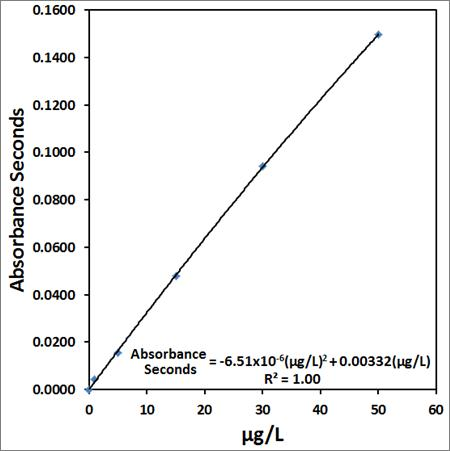Figure 2. Calibration curve for the determination of total arsenic in drinking water by graphite furnace atomic absorption spectroscopy. Concentration is the independent or x variable. The units of concentration are micrograms of arsenic per liter of deionized water (µg/L). Absorbance seconds is the dependent or y variable.

In summary, this calibration obeys the quadratic model (Equation 6; Figure 2). That is, the plot of absorbance seconds versus arsenic concentration is quadratic and the y-intercept goes through the origin.

C.  The Limitations of Using Polynomial Regression to Fit Calibration Curves.

First, a calibration equation is only valid for the detector responses and analyte concentrations that are used to make the curve. Never extrapolate beyond either of these values.

Second, calibration equations must agree with theory or experience. Never use a quadratic calibration equation if theory or experience says the curve should be linear. And never use a cubic calibration equation if theory or experience says the curve should be quadratic. Any deviation from theory or experience suggests that systematic error must be corrected.

Third, this strategy of fitting calibration curves assumes that all errors are random, independent, have a mean of 0, and have a constant variance [4,]. In practice, these assumptions are difficult to test with the relatively few data points that are used in a typical calibration.

Fourth, the algorithms used for polynomial regression are subject to a variety of computational errors . For example, a highly regarded open-source statistical program gave coefficients that are up to 12,000 times greater than those listed in Equations 1 to 6. The calibration results (Tables 1, 2) and regression equations (Equations 1-6) are provided so that readers can test the accuracy of their software. In this study, the regression equations (Equations 1-6) from Microsoft® Excel® 2010 and 2016, MiniTab® 17.2.1, and RStudio® 0.99.441 agreed with each other to at least 3 significant figures.

Table 1. Calibration results from the determination of total arsenic in drinking water by the arsenomolybdate method. The detector measures absorbance. The units of concentration are micrograms of arsenic per liter of deionized water (µg/L).

 Absorbance (µg/L) (µg/L)2 0.000 0 0 0.022 14 196 0.054 28.6 818 0.100 57.1 3,260 0.190 114 12,996 0.386 229 52,441

Table 2. Calibration results from the determination of total arsenic in drinking water by graphite furnace atomic absorption spectroscopy. The detector measures absorbance seconds. The units of concentration are micrograms of arsenic per liter of deionized water (µg/L).

 Absorbance Seconds (µg/L) (µg/L)2 (µg/L)3 0.0000 0 0 0 0.0044 1.0 1 1 0.0156 5.0 25 125 0.0479 15.0 225 3,375 0.0943 30.0 900 27,000 0.1495 50.0 2,500 125,000

4. Conclusions

Polynomial regression gives a simple, reliable, and objective test for linear and curvilinear calibration curves. After this test is finished, a test of the null hypothesis that the y-intercept goes through the origin is used to select the final calibration curve. If the final calibration curve agrees with theory and prior experience, then the instrument is calibrated and is ready to use. If the final calibration curve does not agree with theory and prior experience, then all likely sources of systematic error are corrected and the instrument is recalibrated.

In addition, polynomial regression can be used to determine the linear range of calibration curves for systems that obey Beer’s law. Similarly, polynomial regression can be used to identify detector saturation, the flattening of a calibration curve at high analyte concentrations.

Acknowledgements

This study was supported by Norwich University, Better Life Laboratories, Inc., and Khalifa University.

References

1. WHO (World Health Organization). 2011. Guidelines for Drinking-water Quality. 4th ed. Geneva, Switzerland: World Health Organization.
2. Guidelines Establishing Test Procedures for the Analysis of Pollutants. 2015. 40 CFR Part 136.
3. Frisbie SH, Mitchell EJ, Yusuf AZ, Siddiq MY, Sanchez RE, Ortega R, Maynard DM, Sarkar B. 2005. The development and use of an innovative laboratory method for measuring arsenic in drinking water from western Bangladesh. Environ Health Perspect 113: 1196-1204.
4. Neter J, Wasserman W, Kutner MH. 1985. Applied Linear Statistical Models. 2nd ed. Homewood, IL: Irwin.
5. Buck Scientific, Inc. 2002. Buck Scientific 210VGP Atomic Absorption Spectrophotometer Operator’s Manual. East Norwalk, CT: Buck Scientific, Inc.
6. Skoog, DA, West DM, Holler FJ, Crouch SR. 2014. Fundamentals of Analytical Chemistry. 9th ed. Belmont, CA: Brooks/Cole, Cengage Learning.
7. Bencs L, Szakács O, Szoboszlai N, Ajtony Z, Bozsai G. 2003. Characteristics of atomic absorption calibration curves with transversely heated graphite furnace. J Anal At Spectrom 18: 105-110.
8. Kántor T. 1988. Interpreting some analytical characteristics of thermal dispersion methods used for sample introduction in atomic spectrometry. Spectrochim Acta, Part B 43: 1299-1320.
9. Berglund M, Frech W, Baxter DC, Radziuk B. 1993. A critical evaluation of a multielement ETAAS system using line sources and a transversely heated graphite atomizer with Zeeman effect background correction. Spectrochim Acta, Part B 48: 1381-1392.
10. De Loos-Vollebregt MTC, Van Oosten P, De Koning MJ, Podmos J. 1993. Extension of the dynamic range in a.c. Zeeman electrothermal atomic absorption spectrometry. Spectrochim Acta, Part B 48: 1505-1515.
11. APHA (American Public Health Association), American Water Works Association, Water Environment Federation. 2012. Standard Methods for the Examination of Water and Wastewater. 22nd ed. Washington, DC: American Public Health Association.
12. ISO (International Organization for Standardization). 2001. ISO 8466-2: 2001, Water Quality - Calibration and Evaluation of Analytical Methods and Estimation of Performance Characteristics - Part 2: Calibration Strategy for Non-Linear Second-Order Calibration Functions. Geneva, Switzerland: International Organization for Standardization.
13. Snedecor GW, Cochran WG. 1982. Statistical Methods. 7th ed. Ames, IA: The Iowa University Press.

 Contents 1. 2. 3. 4.
Article ToolsAbstractPDF(381K)